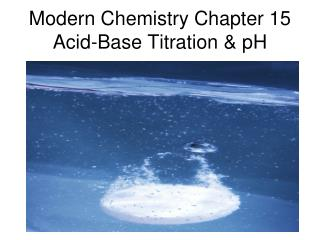DownloadDownload PresentationModern Chemistry Chapter 15 Acid-Base Titration & pH

# Modern Chemistry Chapter 15 Acid-Base Titration & pH

Download Presentation## Modern Chemistry Chapter 15 Acid-Base Titration & pH

- - - - - - - - - - - - - - - - - - - - - - - - - - - E N D - - - - - - - - - - - - - - - - - - - - - - - - - - -
##### Presentation Transcript

1. Modern Chemistry Chapter 15Acid-Base Titration & pH

2. Section 1 • self-ionization of water occurs when two water molecules produce a hydronium (H3O+) and a hydroxide (OH-) ion 2 H2O  H3O+ + OH- • The ionization constant (Kw) of water is: Kw = [H3O+] [OH-] = 1.0 x 10-14 M

3. Acidic, Basic, & Neutral • IF [H3O+] > [OH-] then solution is acidic. • IF [H3O+] < [OH-] then solution is basic. • IF [H3O+] = [OH-] then solution is neutral.

4. Calculating [H3O+] & [OH-] • Since the product of the hydronium and hydroxide ion concentrations is a constant, we can use the following formula to determine these concentrations. [H3O+] [OH-] = 1.0 x 10-14 M Use this formula to answer problems #1-4 on page 502 of the textbook.

5. The pH Scale • The pH of a solution is defined as the negative of the common logarithm of the hydronium ion concentration, [H3O+]. pH = -log [H3O+] • The pOH of a solution is defined as the negative of the common logarithm of the hydroxide ion concentration, [OH-]. pOH = -log [OH-]

6. pH & pOH pH + pOH = 14 so 14 – pH = pOH and 14 – pOH = pH See figure 3 on page on page 503. See table 3 on page 504. See table 4 on page 504. Do problem #1 on page 505. Do problems #1-4 on page 506. Do problems #1-4 on page 508.

7. Cross-Disciplinary Connection • Read the Cross-Disciplinary Connection titled “Liming Streams” on page 510. • Answer both questions at the end of the article.

8. Section 2Determining pH and Titrations • acid-base indicators are compounds whose colors are sensitive to pH • transition interval is the pH range over which an indicator changes color • a pH meter determines the pH of a solution by measuring the voltage between two electrodes placed in a solution.

9. Titration • titration is the controlled addition and measurement of the amount of a solution of a known concentration required to react completely with a measured amount of solution of an unknown concentration • equivalence point is the point at which the two solutions used in a titration are present in chemically equivalent amounts • endpoint is the point in a titration at which the indicator changes color • standard solution is the solution that contains a precisely known concentration of solute

10. Titration • see figure 10 on pages 518-519 for the steps of a titration • A general equation for the solution of titration problems would be VaMaeqa = VbMbeqb Va = volume of acid Vb = volume of base Ma = molarity of acid Mb = molarity of base eqa = #H in formula of acid eqb = #OH in formula of base

11. Titration • Do practice problems #1-2 on page 521. • Do section review problems #1-2 on page 521. • Do chapter review problems #6, 8, 9, 12, 13, 24, 25, & 26 on pages 523-524.

12. Modern Chemistry Chapter 15Test Review • 25 multiple choice • definition of self-ionization • [H3O+] [OH-] = 1 x 10-14 • definition and formula for calculating pH = -log[H3O+] • pH >7 is a base; pH <7 is an acid; pH = 7 is neutral • pH range is normally 0 to 14 • calculate pH from [H3O+] • calculate [H3O+] from pH • definitions of indicators, transition interval, titration, neutralization, endpoint, standard solution • acid-base titration monitors pH • titration problems using VaMaeqa = VbMbeqb